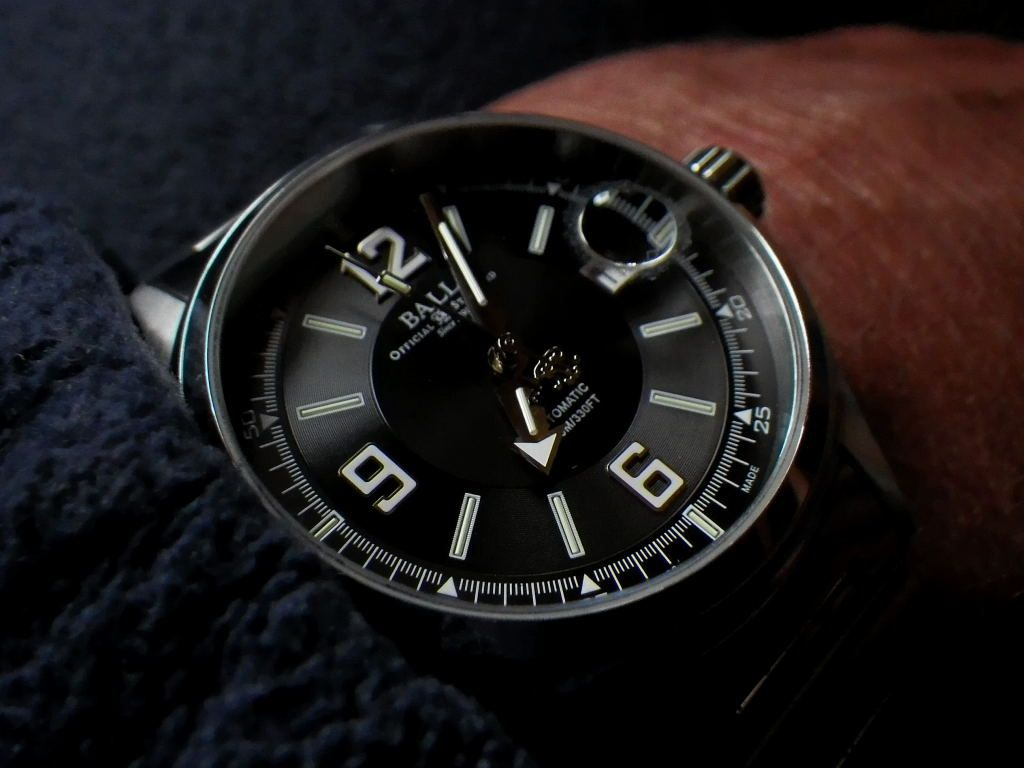((((((((WRUW Thursday 10/20/16))))))))))Thread: ((((((((WRUW Thursday 10/20/16))))))))))

1. ((((((((WRUW Thursday 10/20/16))))))))))Reply With Quote

2. Re: ((((((((WRUW Thursday 10/20/16))))))))))Reply With Quote

3. Re: ((((((((WRUW Thursday 10/20/16))))))))))Reply With Quote

4.

5. Re: ((((((((WRUW Thursday 10/20/16))))))))))Reply With Quote

6. Re: ((((((((WRUW Thursday 10/20/16))))))))))Reply With Quote

7. Re: ((((((((WRUW Thursday 10/20/16))))))))))

Arrived yesterday afternoon. My first JDM. Here she is! (my weak photo skills notwithstanding)Reply With Quote

8. Re: ((((((((WRUW Thursday 10/20/16))))))))))Reply With Quote

9. Re: ((((((((WRUW Thursday 10/20/16))))))))))Reply With Quote

10. Re: ((((((((WRUW Thursday 10/20/16))))))))))

Nothing special at a glance but..............I've been wearing that since September 31st and mean to finish out October with it. Wear habit is 24/7 and that watch is currently -1 when compared to Time dot Gov. from when it was set 21 days ago!Reply With Quote

11. Re: ((((((((WRUW Thursday 10/20/16))))))))))Background Vogue Hommes

Sent from my iPhone using TapatalkReply With Quote